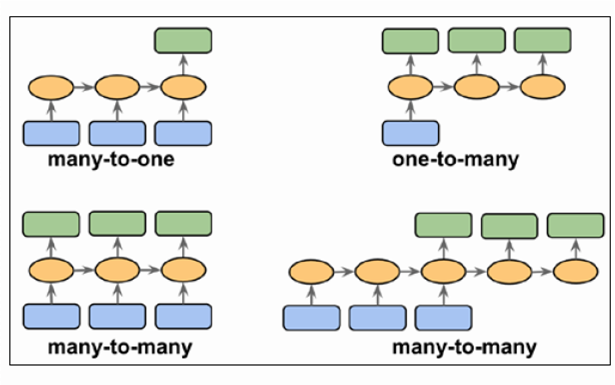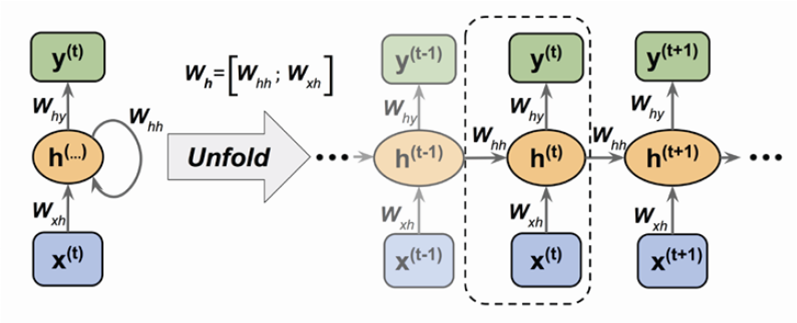## 2019/1/23

### Recurrent Neural Networks (RNN) 原理 1/2

27 Recurrent Neural Networks (RNN) 原理 1/2

Recurrent Neural Networks (RNN) 中文可稱為遞迴神經網路，在一般的順向神經網路（如CNN）輸入的data是經一級再一級單向依序處理，最開始是輸入級，最後是輸出級，每一筆data和前後輸入的data間都沒有任何關聯性，例如在處理MINST 資料集時每筆輸入的data代表一張手寫數字圖，各筆之間是無任何關係的。但在遞迴神經網路中考慮的data是前後有相關的，很多現實應用中的data都是具有此種特性，如語音或影片。有此特性的data若用順向神經網路處理將無法攫取出data前後的關聯性。

RNN的一個神經元有時又叫cell （晶元），此種cell可視成具有記憶，亦即會記住先前一段時間前所得到的結果，或稱state（狀態），也可視為具有feedback (回授或回饋）。但是要記住的「先前一段時間」是要多久呢？這是一個重要的考量問題，理論上是啊記住先前無限長的時間，但是實際處理的考量因需記憶的狀態以及相關的係數必需是有限的，這有限的時間長度有時稱為記憶的深度(deep)，所以有很多「深度」必需釐清，例如CNN 中layers的數目也叫深度，一張圖像的色彩空間 (如R,G,B)也叫深度。

► Unrolling a cell （解開晶元)Unrolling a cell

► Different sequence modeling (不同的序列模型化）Different sequence modeling

• Many-to-one (多至一）: 輸入是一個序列的向量，但輸出只有一個向量 ，例如在語意分析的應用，輸入是一串文字，而輸出是一向量表示不同的語意或等級，如五星中的幾星。
• One-to-many（一至多）:輸入是一個固定的格式，但輸出是一個序列的向量，例如在圖像的應用中，可依輸入固定格式的圖片產生序列向量的標題。
• Synchronized many-to-many（同步多至多）: 如上圖左下小圖，輸入和輸出的序列是同步的，這也是我們在 Unrolling a cell 圖中所示的情形，例如在視訊訊框的分類應用中，我們可對每個frame (訊框) 分類。
• Delayed many-to-man （延遲多至多）:一序列的輸入經過幾個時階的延遲後會有一序列的輸出，例如在語言翻譯中，需經過某些時後才能得知整個句子的語意，也才能有輸出。
► RNN 架構的幾個重要觀念：
• cell 的記憶深度：這和輸入data block 長度有關，是長度減1，因常常以解開的情形表示一個cell，所以data block 長度就是時階的長度。
• input vector: 指的是一筆輸入的向量，向量有其寬度，也就是輸入的寬度。
• state vector:  指的是隱藏狀態的向量，向量有其寬度，也就是隱藏狀態的寬度
• mini-batch (or batch): 指一次批量處理多少筆輸入向量，若是 n 筆，則在該層就需有n 個cell (neurons) 並列，這些並列的 neurons 各有自己的權重係數，並行輸出，彼此無關。
• layer: 這和在順向網路的層是一樣的，也就是上一層的輸出再交給下一層的輸入。這和解開的cell中表示的訙流動的意義是不一樣的，解開中是針對在一個層裡的一個cell，雖然解開成很多時階串接，但實際上只有一組權重。在不同 layers的情況則不一樣，因每個cell是獨立運作，所以有各自的權重。若是多層的網路依時階解開則會是二維圖形，一維是時階，另一維是層次。
► 多層unfolded 圖多層時解開圖

► 單一晶元權值 (Weighting)係數權重係數圖

$\boldsymbol{h}^{(t)}=\phi _h\left ( \boldsymbol{W}_{xh}\boldsymbol{x}^{(t)}+\boldsymbol{W}_{hh} \boldsymbol{x}^{(t-1)}+\boldsymbol{b}_{h}\right )=\phi _h\left (\left [ \boldsymbol{W}_{xh};\boldsymbol{W}_{hh} \right ] \begin{bmatrix} \boldsymbol{x}^{(t)}\\ \boldsymbol{h}^{(t-1)} \end{bmatrix} +\boldsymbol{b}_{h}\right )$
$\phi _h$ 為隱藏狀態的作動函數 ，而輸出$\boldsymbol{y}^{(t)}$可寫成
$\boldsymbol{y}^{(t)}=\phi _y\left ( \boldsymbol{W}_{hy}\boldsymbol{h}^{(t)}+\boldsymbol{b}_{y} \right )$
$\phi _y$為輸出的作動函數。

RNN 的權值更新是使用 Backpropagation through time (BPTT) 的方法，也就是利用誤差後向傳播於解開的模型，基本原理和我們在  Artificial Neural Networks (ANN, 人工神經網路) 單元討論的都一樣，其中一差別是在BPTT 中隱藏狀態有兩個輸入，一是前一時階隱藏狀態輸出，一是當前時階的輸入，所以係數更新求偏導時這兩輸入的方向都要考量，另一差別是相同名稱的係數在每一時階都是同一係數（只是我們將其展開而已，實際上一名稱就只有一個係數存在），所以需將所需更新的值從當前輸出的誤差一路向後求偏微，並將同一係數需更新的值累加，最後再進行更新。

► TensorFlow 例子 1 from scratch

import numpy as np
import tensorflow as tf
import matplotlib.pyplot as plt
tf.reset_default_graph()
tf.set_random_seed(101)
np.random.seed(101)
n_inputs = 3
n_neurons = 5
X0 = tf.placeholder(tf.float32, [None, n_inputs])
X1 = tf.placeholder(tf.float32, [None, n_inputs])
Wx = tf.Variable(tf.random_normal(shape=[n_inputs, n_neurons],dtype=tf.float32))
Wy = tf.Variable(tf.random_normal(shape=[n_neurons,n_neurons],dtype=tf.float32))
b = tf.Variable(tf.random_normal([1, n_neurons], dtype=tf.float32))
Y0 = tf.tanh(tf.matmul(X0, Wx) + b)
Y1 = tf.tanh(tf.matmul(Y0, Wy) + tf.matmul(X1, Wx) + b)
init = tf.global_variables_initializer()
X0_batch = np.array([[0, 1, 2], [3, 4, 5], [6, 7, 8], [9, 0, 1]]) # t = 0
X1_batch = np.array([[9, 8, 7], [0, 0, 0], [6, 5, 4], [3, 2, 1]]) # t = 1
with tf.Session() as sess:
init.run()
Y0_val, Y1_val = sess.run([Y0, Y1], feed_dict={X0: X0_batch, X1: X1_batch})
print(Y0_val)
print(Y1_val)

[[ 0.9937752   0.70438135 -0.99904335 -0.985441   -0.9923303 ]
[ 0.999982    0.7793329  -1.         -0.99999994 -1.        ]
[ 1.          0.83709735 -1.         -1.         -1.        ]
[-0.746156   -0.9999984  -0.935545   -0.99999875  0.99992156]]
[[ 0.99999964 -0.22571692 -0.9999341  -1.         -1.        ]
[ 0.32428783  0.7286062   0.9998977  -0.9945999  -0.01352311]
[ 0.99986684 -0.31827345 -0.3926238  -1.         -1.        ]
[ 0.9612457   0.73960024 -0.96762794 -0.6256297   0.84796953]]

► TensorFlow 例子2 使用靜態cell

import numpy as np
import tensorflow as tf
import matplotlib.pyplot as plt
tf.reset_default_graph()
tf.set_random_seed(101)
np.random.seed(101)
n_inputs = 3
n_neurons = 5

X0 = tf.placeholder(tf.float32, [None, n_inputs])
X1 = tf.placeholder(tf.float32, [None, n_inputs])
X0_batch = np.array([[0, 1, 2], [3, 4, 5], [6, 7, 8], [9, 0, 1]]) # t = 0
X1_batch = np.array([[9, 8, 7], [0, 0, 0], [6, 5, 4], [3, 2, 1]]) # t = 1
basic_cell = tf.keras.layers.SimpleRNNCell(units=n_neurons)
output_seqs, states = tf.nn.static_rnn(basic_cell, [X0, X1],
dtype=tf.float32)
Y0, Y1 = output_seqs
init = tf.global_variables_initializer()
with tf.Session() as sess:
init.run()
Y0_val, Y1_val = sess.run([Y0, Y1], feed_dict={X0: X0_batch, X1: X1_batch})

print(Y0_val)
print(Y1_val)

[[-0.5652171   0.5697026   0.7122883  -0.8409974   0.88083786]
[-0.9912105  -0.8787464   0.9957598  -0.99976444  0.999285  ]
[-0.99985975 -0.99771893  0.99994624 -0.99999964  0.99999607]
[-0.45131958 -0.99999744  0.995488   -0.766692    0.9992609 ]]
[[-0.99999475 -0.99999785  0.99997574 -1.          0.9999992 ]
[-0.89276123  0.7036167   0.24479836 -0.01462305  0.8770031 ]
[-0.99990094 -0.9988354   0.9988652  -0.9999509   0.9999356 ]
[-0.98935765 -0.9510143   0.79948956 -0.9464767   0.9857446 ]]


► TensorFlow 例子3 靜態cell with list input

import numpy as np
import tensorflow as tf
import matplotlib.pyplot as plt
tf.reset_default_graph()
tf.set_random_seed(101)
np.random.seed(101)
n_steps = 2
n_inputs = 3
n_neurons = 5
X = tf.placeholder(tf.float32, [None, n_steps, n_inputs])
X_seqs = tf.unstack(tf.transpose(X, perm=[1, 0, 2]))
basic_cell = tf.keras.layers.SimpleRNNCell(units=n_neurons)
output_seqs, states = tf.nn.static_rnn(basic_cell, X_seqs,
dtype=tf.float32)
outputs = tf.stack(output_seqs)
X_batch = np.array([
[[0, 1, 2], [9, 8, 7]],
[[3, 4, 5], [0, 0, 0]],
[[6, 7, 8], [6, 5, 4]],
[[9, 0, 1], [3, 2, 1]],
])
init = tf.global_variables_initializer()
with tf.Session() as sess:
init.run()
outputs_val = sess.run (outputs,feed_dict={X: X_batch})
print(outputs_val)

[[[-0.6306404   0.55795866 -0.7999877   0.21747808  0.07178839]
[-0.9948304   0.55989635 -0.98583406  0.7085942   0.9573955 ]
[-0.99994075  0.5618281  -0.9990845   0.9134054   0.9989066 ]
[-0.99998313 -0.9999837   0.5767984   0.99884075  0.9475927 ]]

[[-0.99989617 -0.7658852  -0.9972035   0.89165974  0.99996704]
[ 0.9162878  -0.19949536  0.1659006  -0.74881357 -0.44842872]
[-0.97042847 -0.6989903  -0.89608663  0.13549285  0.99437046]
[-0.9118176   0.31645307 -0.34153438 -0.5685557   0.30243096]]]


► TensorFlow 例子4 動態cell

import numpy as np
import tensorflow as tf
import matplotlib.pyplot as plt
tf.reset_default_graph()
tf.set_random_seed(101)
np.random.seed(101)
n_steps = 2
n_inputs = 3
n_neurons = 5
X = tf.placeholder(tf.float32, [None, n_steps, n_inputs])
basic_cell = tf.keras.layers.SimpleRNNCell(units=n_neurons)
outputs, states = tf.nn.dynamic_rnn(basic_cell, X, dtype=tf.float32)
init = tf.global_variables_initializer()
X_batch = np.array([
[[0, 1, 2], [9, 8, 7]],
[[3, 4, 5], [0, 0, 0]],
[[6, 7, 8], [6, 5, 4]],
[[9, 0, 1], [3, 2, 1]],
])
with tf.Session() as sess:
init.run()
outputs_val = outputs.eval(feed_dict={X: X_batch})
print(outputs_val)

[[[ 0.29776108  0.10881581  0.9179363   0.6650894  -0.0652646 ]
[ 0.9998412   0.61094993  1.         -0.99996066 -1.        ]]

[[ 0.97577417  0.37916356  0.9999975  -0.50007206 -0.99473137]
[-0.8628299  -0.10816464  0.6300342  -0.5194082   0.6967999 ]]

[[ 0.9994444   0.5972857   1.         -0.9562965  -0.9999841 ]
[ 0.97939676  0.47597754  1.         -0.9993922  -0.99973005]]

[[ 0.9999989   0.9990174   0.9999999  -0.99998325 -0.9999981 ]
[ 0.5214528   0.19148043  0.9998562  -0.99468046 -0.8851629 ]]]


► Vanishing and exploding gradient problem （梯度消失和爆表問題）
求神網路的後向傳播時必需由輸出級向後求梯度至輸入級，因鏈鎖律的關係，所求出的梯度必需一路向後相乘，因而會呈指數成長或衰退。當神經網路時的深度很深時（RNN 解開模型中，長時階後向傳播也是一種深度很深的網路）如果偏微值小 (<1), 則連乘後會逐漸消失導致無法學習 ,這稱為梯度消失問題，當偏值大時 (>1) 則一路相乘的結果會造成爆表，導致模型崩解。在RNN中，為了解決此兩問題，有各種不同的變型cell 被提出，下一單元會探討一些變型的RNN cell。

Aurélien Géron, Hands-On Machine Learning with Scikit-Learn and TensorFlow, O'Reilly, 2017